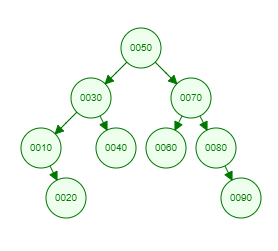Recent Tutorials and Articles
Implementing Level Order (Breadth First) Traversal of Binary Tree in Java
Published on: 27th October 2018

This tutorial provides code for implementing level order (breadth first) traversal of a Binary Tree.

# Level Order Traversal or Breadth First Search (BFS) of Binary Tree

Binary Tree traversal is a process of visiting each node of Binary Tree exactly once. Level Order traversal falls into Breadth first search category as it moves through all the siblings of a node before moving it to its children.

Breadth first search is very useful in algorithms used for finding friends at specified distance in social networking, finding shortest distance, search crawlers and finding all neighbour locations in GPS applications.

Let's consider below Binary Tree -Here is the level order traversal  result of above tree -

``````50
30 70
10 40 60 80
20 90
``````

# Java Implementation of Level Order Traversal of Binary Tree

Here is Java code for Binary Tree containing methods for inserting data and level order traversal -

``````package com.aksain.data.structures;

import java.util.Queue;

public class BinaryTreeWithLevelOrderTraversal<T extends Comparable<T>> {
private Node root;

private class Node {
T data;
Node left;
Node right;

private Node(T data) {
this.data = data;
}
}

private Node doInsert(T data, Node node) {
if(node == null) {
return new Node(data);
}
int result = data.compareTo(node.data);
if(result < 0) {
node.left = doInsert(data, node.left);
} else if(result > 0) {
node.right = doInsert(data, node.right);
}

return node;
}

public void insert(T data) {
root = doInsert(data, root);
}

private void doLevelOrderTraversal(Node root) {
final Queue<Node> queue = new LinkedList<>();

while(!queue.isEmpty()) {
int noOfElementsAtThisLevel = queue.size();
for(int index = 0; index < noOfElementsAtThisLevel; index++) {
Node tmp = queue.poll();
System.out.print(tmp.data + " ");
if(tmp.left != null) {
}
if(tmp.right != null) {
}
}
System.out.println();
}
}

public void levelOrderTraversal() {
doLevelOrderTraversal(root);
}

public static void main(String[] args) {
final BinaryTreeWithLevelOrderTraversal<Integer> binaryTree = new BinaryTreeWithLevelOrderTraversal<>();
binaryTree.insert(50);
binaryTree.insert(30);
binaryTree.insert(10);
binaryTree.insert(20);
binaryTree.insert(40);
binaryTree.insert(70);
binaryTree.insert(60);
binaryTree.insert(80);
binaryTree.insert(90);

binaryTree.levelOrderTraversal();
}
}
``````

Here is the output of above code -

``````50
30 70
10 40 60 80
20 90
``````

Thank you for reading through the tutorial. In case of any feedback/questions/concerns, you can communicate same to us through your comments and we shall get back to you as soon as possible.

Published on: 27th October 2018